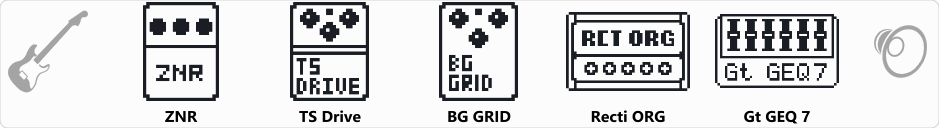# 7 String Rhythm Tone

Discussion in 'Zoom G3n/G3Xn/G5n' started by jriff79, Dec 19, 2019.

1. 7 String Rhythm ToneDevice: Zoom G3n
Firmware: 2.20

Name on device: 7 STRING
Optimized for: Guitar Amp

Effects chain:7 String Rhythm Tone. Best if used with real 4x12 cabinet or modeling amp with amp turned off and only the cabinet model turned on such as a Line 6 Spider V. Utilizing the amp on the Zoom G3N and the cabinet on the external amp.

Effect: "ZNR" (Dynamics), active - "yes"
"Detect" = GTRIN
"Depth" = 100
"Threshold" = 100
"Decay" = 0

Effect: "TS Drive" (Overdrive / Distortion), active - "yes"
"Gain" = 100
"Boost" = On
"Tone" = 50
"Volume" = 50

Effect: "BG GRID" (Overdrive / Distortion), active - "yes"
"Gain" = 100
"Tone" = 50
"Balance" = 50
"Volume" = 49

Effect: "Recti ORG" (Amp simulator), active - "yes"
"Mode" = MDRN
"Bass" = 0
"Middle" = 50
"Treble" = 100
"Presence" = 85
"Gain" = 100
"Volume" = 56
"SOLO" = 1

Effect: "Gt GEQ 7" (Filter), active - "yes"
"100 Hz" = 0.0
"200 Hz" = 0.0
"400 Hz" = 0.0
"800 Hz" = 0.0
"1.6 kHz" = 0.0
"3.2 kHz" = 0.0
"6.4 kHz" = 11.5
"Volume" = 80
"100 Hz" = 0.0
"200 Hz" = 0.0
"400 Hz" = 0.0
"800 Hz" = 0.0
"1.6 kHz" = 2.0
"3.2 kHz" = 11.5
"6.4 kHz" = 12.0
"Volume" = 89
"Parameter set" = A
Patch Volume: 13